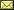# RGMIA Monographs

Z. TomovskiConvergence and Integrability on some Classes of Trigonometric Series
(86 pages in total)

• Contents and abstract   (ps) (pdf

• Chapters I - II, pages 1 - 42   (ps) (pdf

• Chapters III - IV and References, pages 43 - 86   (ps) (pdf

Abstract

In this work, the  theory of L1-convergence  on some classes of trigonometric series is elaborated. It contains  four chapters in which some new results are obtained. Also, new proofs of  some well-known theorems are given. A classical result concerning the  integrability and L1 convergence of a cosine series
 ¥ a0 / 2 + å an cos nx (C) n=1

with convex coefficients is the well-known theorem of Young .

Later, Kolmogorov   extends Young's result for series (C) with quasiconvex coefficients and also showed that such cosine series converge in L1-norm if and only if

an log n = o(1), as n ® ¥.

In 1973, S. A. Telyakovskii  extended the old classical result of Kolmogorov. Namely, Telyakovskii introduced a Sidon-type condition  described by class S in  his paper. Firstly, he proved that the Sidon's class is equivalent to  the class S, and second that S is a  L1-integrability class for series (C). Also,  Telyakovskii in his paper showed that such cosine series (C) converge in L1-norm iff

an log n = o(1),  n ® ¥

The class S is  usually called as Sidon-Telyakovskii class. Several authors, Boas, Fomin, C. Stanojevic, Bojanic, and others have extended these  classical results by answering one or both of the following two questions:

(i) If {an} belongs to the class of null sequences of bounded  variation BV, then (C) is the Fourier series of its sum  function f.

(ii) If {an}Î BV, then (C) is the  Fourier series of some function f Î L1 and  || Sn - f || = o(1),  as n ® iff an log n = o(1), n ®. Here,  Sn denotes the n-th partial sum of the series (C), and  || · || is the L1-norm. Namely, these authors, for any  classes of sequences have  given a positive answer of the question (ii). Then we have considered the  problem of L1-convergence of the r-th  derivate of Fourier series, i.e. we defined some new integrability  classes of  the r-th derivate of the Fourier series. Some necessary and sufficient conditions for L1-convergence of the r-th derivate of Fourier series are obtained. Also it is  shown a version of Uljanov's theorem  and extend it to the r-th derivate of trigonometric series.